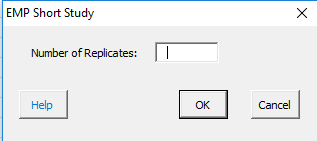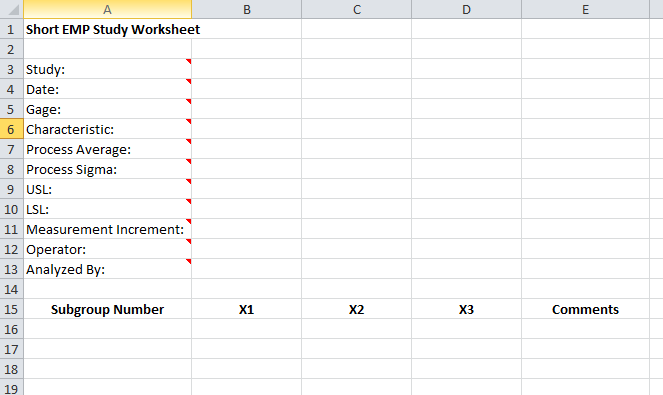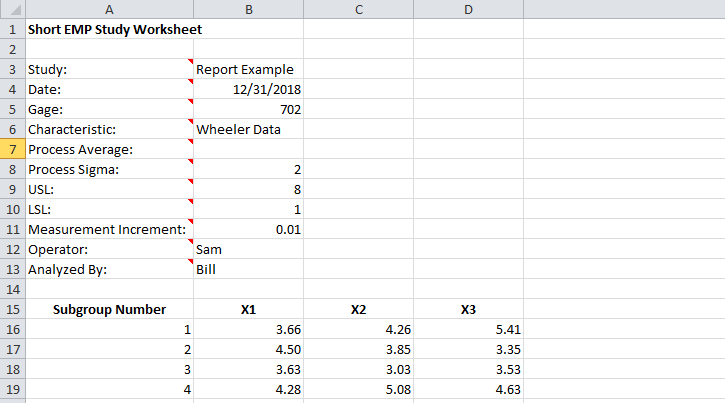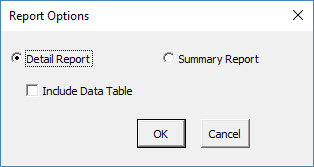# Short EMP Study HelpIn a Short EMP Study, one operator measures multiple parts using one measurement system.  The results are then analyzed using different approaches including a X-R chart.  The subgroups are formed based on the results from one part.  The part to part variation is shown on the X chart.  The within part variation from the range chart,  is a measure of the measurement error.  The limits on the X chart represent this measurement error so you want to have out of control points on the X chart.  If the parts represent production, you can determine how much of the total variance is due to the measurement system.  If they are not representative of production, you can use an historical measure of variation to estimate the effect of the measurement system on total variance.  This help page describes how to perform the EMP consistency study using data that be downloaded at this link.

1. To start the analysis, select “MSA” from the “Analysis” panel on the SPC for Excel ribbon.  Then select “EMP” and “Short EMP Study”.  The input form below is shown. Enter the number of replicates and select OK.2. A Short EMP Study worksheet is added to the workbook. This is where you enter the information.3. Enter the information into the top part of the worksheet.  There is a comment in each cell in rows 3 to 13 in column A that explain the entry.  The only required entry is the measurement increment.  Each entry is explained below.

• Study: optional, name of study
• Date: optional, date short EMP performed
• Gage: optional, name of gage
• Characteristic: optional, characteristic being measured
• Process Average: optional, if entered used to calculate Cpk for one-sided specs for the class of monitor
• Process Sigma: optional, if entered used to calculate the total variance instead of using the total variance from the data
• USL: optional, if entered used to calculate the Cp/Cpk values for the class of monitor; Cp if both specs are entered, Cpk if one spec is entered
• LSL: optional, if entered used to calculate the Cp/Cpk values for the class of monitor; Cp if both specs are entered, Cpk if one spec is entered
• Measurement Increment: required, used to determine if measurement increment is adequate based on probable error; also used to determine manufacturing specs if specs are entered
• Operator: optional, name of operator running the tests
• Analyzed By: optional, name of person doing the analysis

4. Enter the subgroup number, the sample results for each replication and any comments (optional).

5. Part of a completed short EMP worksheet is shown below.6. The results are now ready to be analyzed using the EMP consistency method. To analyze the results, select “MSA” from the “Analysis” panel on the SPC for Excel ribbon.

7. The following options are displayed.  You have an option for detailed report or a summary report, both displayed on single worksheet. The detailed report contains all the charts, calculations and explanation of results.  The summary report contains the charts.  You also have the option to include a data table in the report.  Select OK to generate the report in the workbook.8. A pdf file containing the detailed report with a description of the output is given at this link.  The output includes:

•  X-R chart
• Control chart calculations
• X chart analysis
• R chart analysis
• Repeatability (test-retest error)
• Probable error and measurement increment
• Watershed specifications and precision to tolerance ratio
• Overall variance and product variance
• Intraclass correlation coefficient
• Type of Class Monitor
• Data table